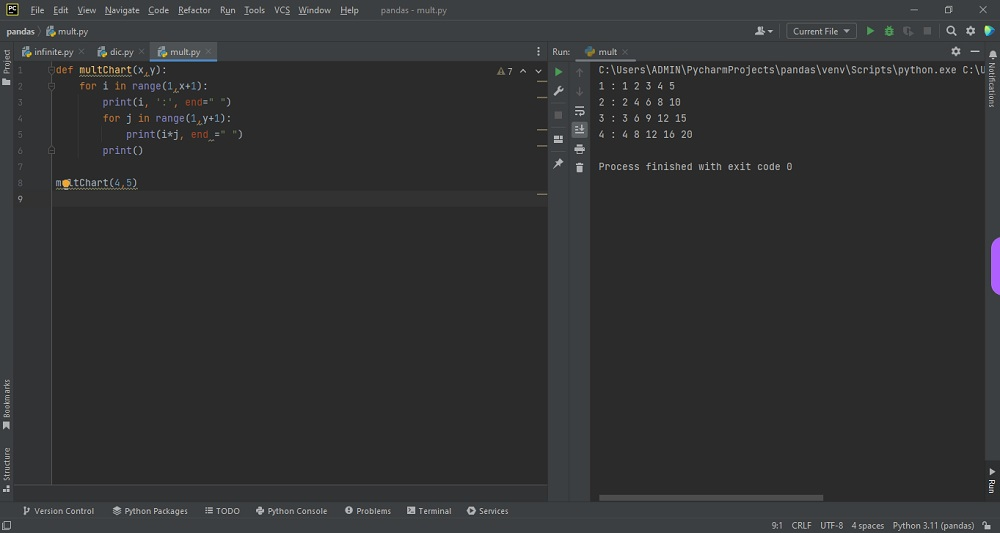# How to Print Multiplication Table based on Two Inputs in Pythonby vsasikalabeUpdated: Jan 31, 2023Guide Kit

An algebraic system's multiplication operation is defined in a multiplication table, which is a mathematical table. It lists the product of a number (known as the multiplicand) and all the integers from 1 to a given value (known as the range of the table).

Each row of the table represents the product of a particular number (the multiplicand), and each column represents the product of that number and a particular multiplier. The intersection of a row and column gives the product of the multiplicand and multiplier. The table is usually arranged in a grid format with the multiplicand on one axis and the multiplier on the other. The number for which you wish to discover the multiplication table may be iterated through using a for loop, and inside that loop, another for loop can be used to iterate through the range of the first input (the number of times you want to multiply the second input by). At each iteration, print the second input’s result multiplied by the inner loop’s current iteration number.

You may have a look at the code given below for printing multiplication table based on two inputs.Fig : Preview of the output that you will get on running this code from your IDE.

### Instructions

Follow the steps carefully to get the output easily.

2. Open new python file on your IDE.
3. Copy the snippet using the 'copy' and paste it in your python file.
4. Run the file to generate the output.

I hope you found this useful.

I found this code snippet by searching for ' Print multiplication table based on two inputs'in kandi. You can try any such use case!

### Environment Tested

I tested this solution in the following versions. Be mindful of changes when working with other versions.

1. The solution is created in PyCharm 2021.3.
2. The solution is tested on Python 3.9.7.

Using this solution, we are able to Print multiplication table based on two inputs using python with simple steps. This process also facilities an easy way to use, hassle-free method to create a hands-on working version of code which would help us to Print multiplication table based on two inputs using python.

### Support

1. For any support on kandi solution kits, please use the chat
2. For further learning resources, visit the Open Weaver Community learning page